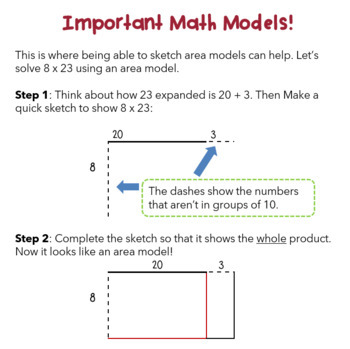4th - 5th
Subjects
Standards
Resource Type
Formats Included
• PDF
Pages
126 pages

### Description

Boost your Fourth Grader's math concepts and strategies with our 6-week skill building packet!

This engaging set of of student and parent-friendly resources blends critical thinking activities, games, and practice pages to keep kids learning all summer long!

This complete curriculum address all the critical CCSS-M standards for 4th Grade, including:

• Place Value to the millions
• Multiplication with multiple models
• The standard algorithm for multiplication
• Understanding Division through multiple models
• Area models, arrays, and ratio tables
• Fractions and decimals
• Lines, angles, and polygons
• Real world story problems

We have summer packets for Grades K-4, with Grade 5 coming out soon! Check out our TpT store for all our curriculum!

Total Pages
126 pages
N/A
Teaching Duration
N/A
Report this Resource to TpT
Reported resources will be reviewed by our team. Report this resource to let us know if this resource violates TpT’s content guidelines.

### Standards

to see state-specific standards (only available in the US).
Interpret a multiplication equation as a comparison, e.g., interpret 35 = 5 × 7 as a statement that 35 is 5 times as many as 7 and 7 times as many as 5. Represent verbal statements of multiplicative comparisons as multiplication equations.
Multiply or divide to solve word problems involving multiplicative comparison, e.g., by using drawings and equations with a symbol for the unknown number to represent the problem, distinguishing multiplicative comparison from additive comparison.
Solve multistep word problems posed with whole numbers and having whole-number answers using the four operations, including problems in which remainders must be interpreted. Represent these problems using equations with a letter standing for the unknown quantity. Assess the reasonableness of answers using mental computation and estimation strategies including rounding.
Find all factor pairs for a whole number in the range 1-100. Recognize that a whole number is a multiple of each of its factors. Determine whether a given whole number in the range 1-100 is a multiple of a given one-digit number. Determine whether a given whole number in the range 1-100 is prime or composite.
Generate a number or shape pattern that follows a given rule. Identify apparent features of the pattern that were not explicit in the rule itself. For example, given the rule “Add 3” and the starting number 1, generate terms in the resulting sequence and observe that the terms appear to alternate between odd and even numbers. Explain informally why the numbers will continue to alternate in this way.